The objective of this experimental study is to obtain the data on pressure loss characteristics of the fuel assembly which must be deformed during hypothetical core disruptive accident (HCDA). Three of deformed bundle and one of normal shaped bundle were used in the experiment. These bundles had the same geometry as the fuel bundle part of "Monju" blanket assembly. The main conclusions are as follows: The pressure loss (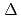P) of deformed bundle at the same Reynolds numbers as normal bundle can approximately predicted by using the ratio of cross sectional flow area(A), as shown below. (P(deformed))/((normal)) = (A(normal)/A(deformed))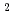. This equation is applicable with the following limitations. (1)Reynolds numbers defined at bundle area is 10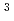to 3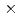10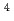. (2)Reduction of flow area is within 17% of flow area of normal bundle. Prediction area of the equation is less than 8% and 3% for the range of Reynolds number of 10to 810and 810to 310, respectively.